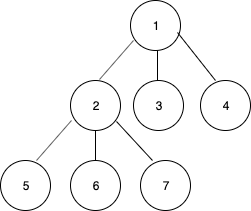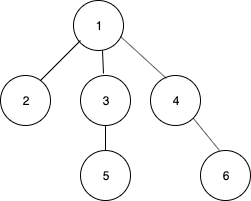# Inorder traversal of an N-ary Tree

Given an N-ary tree containing, the task is to print the inorder traversal of the tree.

Examples:

Input: N = 3Output: 5 6 2 7 3 1 4

Input: N = 3Output: 2 5 3 1 4 6

## Recommended: Please try your approach on {IDE} first, before moving on to the solution.

Approach: The inorder traversal of an N-ary tree is defined as visiting all the children except the last then the root and finally the last child recursively.

• Recursively visit the first child.
• Recursively visit the second child.
• …..
• Recursively visit the second last child.
• Print the data in the node.
• Recursively visit the last child.
• Repeat the above steps till all the nodes are visited.

Below is the implementation of the above approach:

## C++

 `// C++ implementation of the approach ` `#include ` `using` `namespace` `std; ` ` `  `// Class for the node of the tree ` `struct` `Node  ` `{ ` `    ``int` `data; ` ` `  `    ``// List of children ` `    ``struct` `Node **children; ` `     `  `    ``int` `length; ` `     `  `    ``Node() ` `    ``{ ` `        ``length = 0; ` `        ``data = 0;  ` `    ``} ` ` `  `    ``Node(``int` `n, ``int` `data_) ` `    ``{ ` `        ``children = (Node**)``malloc``(``sizeof``(Node*)*n); ` `        ``length = n; ` `        ``data = data_; ` `    ``} ` `}; ` ` `  `// Function to print the inorder traversal ` `// of the n-ary tree ` `void` `inorder(Node *node) ` `{ ` `    ``if` `(node == NULL) ` `        ``return``; ` ` `  `    ``// Total children count ` `    ``int` `total = node->length; ` `     `  `    ``// All the children except the last ` `    ``for` `(``int` `i = 0; i < total - 1; i++) ` `        ``inorder(node->children[i]); ` ` `  `    ``// Print the current node's data ` `    ``cout<< node->data << ``" "``; ` ` `  `    ``// Last child ` `    ``inorder(node->children[total - 1]); ` `} ` ` `  `// Driver code ` `int` `main() ` `{ ` ` `  `    ``/* Create the following tree  ` `            ``1 ` `            ``/ | \ ` `        ``2 3 4 ` `        ``/ | \ ` `        ``5 6 7 ` `    ``*/` `    ``int` `n = 3; ` `    ``Node* root = ``new` `Node(n, 1); ` `    ``root->children = ``new` `Node(n, 2); ` `    ``root->children = ``new` `Node(n, 3); ` `    ``root->children = ``new` `Node(n, 4); ` `    ``root->children->children = ``new` `Node(n, 5); ` `    ``root->children->children = ``new` `Node(n, 6); ` `    ``root->children->children = ``new` `Node(n, 7); ` ` `  `    ``inorder(root); ` `    ``return` `0; ` `} ` ` `  `// This code is Contributed by Arnab Kundu `

## Java

 `// Java implementation of the approach ` `class` `GFG { ` ` `  `    ``// Class for the node of the tree ` `    ``static` `class` `Node { ` `        ``int` `data; ` ` `  `        ``// List of children ` `        ``Node children[]; ` ` `  `        ``Node(``int` `n, ``int` `data) ` `        ``{ ` `            ``children = ``new` `Node[n]; ` `            ``this``.data = data; ` `        ``} ` `    ``} ` ` `  `    ``// Function to print the inorder traversal ` `    ``// of the n-ary tree ` `    ``static` `void` `inorder(Node node) ` `    ``{ ` `        ``if` `(node == ``null``) ` `            ``return``; ` ` `  `        ``// Total children count ` `        ``int` `total = node.children.length; ` `        ``// All the children except the last ` `        ``for` `(``int` `i = ``0``; i < total - ``1``; i++) ` `            ``inorder(node.children[i]); ` ` `  `        ``// Print the current node's data ` `        ``System.out.print(``""` `+ node.data + ``" "``); ` ` `  `        ``// Last child ` `        ``inorder(node.children[total - ``1``]); ` `    ``} ` ` `  `    ``// Driver code ` `    ``public` `static` `void` `main(String[] args) ` `    ``{ ` ` `  `        ``/* Create the following tree  ` `                   ``1 ` `                ``/  |  \ ` `               ``2   3   4 ` `             ``/ | \ ` `            ``5  6  7 ` `        ``*/` `        ``int` `n = ``3``; ` `        ``Node root = ``new` `Node(n, ``1``); ` `        ``root.children[``0``] = ``new` `Node(n, ``2``); ` `        ``root.children[``1``] = ``new` `Node(n, ``3``); ` `        ``root.children[``2``] = ``new` `Node(n, ``4``); ` `        ``root.children[``0``].children[``0``] = ``new` `Node(n, ``5``); ` `        ``root.children[``0``].children[``1``] = ``new` `Node(n, ``6``); ` `        ``root.children[``0``].children[``2``] = ``new` `Node(n, ``7``); ` ` `  `        ``inorder(root); ` `    ``} ` `} `

## Python3

 `# Python3 implementation of the approach ` `class` `GFG: ` `     `  `    ``# Class for the node of the tree ` `    ``class` `Node: ` `        ``def` `__init__(``self``,n,data): ` `            ``# List of children ` `            ``self``.children ``=` `[``None``]``*``n ` `            ``self``.data ``=` `data ` `     `  `    ``# Function to print the inorder traversal  ` `    ``# of the n-ary tree  ` `    ``def` `inorder(``self``, node): ` `        ``if` `node ``=``=` `None``: ` `            ``return` `         `  `        ``# Total children count  ` `        ``total ``=` `len``(node.children) ` `         `  `        ``# All the children except the last ` `        ``for` `i ``in` `range``(total``-``1``): ` `            ``self``.inorder(node.children[i]) ` `         `  `        ``# Print the current node's data ` `        ``print``(node.data,end``=``" "``) ` `         `  `        ``# Last child  ` `        ``self``.inorder(node.children[total``-``1``]) ` `     `  `    ``# Driver code ` `    ``def` `main(``self``): ` `        ``# Create the following tree   ` `        ``#          1  ` `        ``#       /  |  \  ` `        ``#      2   3   4  ` `        ``#    / | \  ` `        ``#   5  6  7 ` `         `  `        ``n ``=` `3` `        ``root ``=` `self``.Node(n, ``1``) ` `        ``root.children[``0``] ``=` `self``.Node(n, ``2``) ` `        ``root.children[``1``] ``=` `self``.Node(n, ``3``) ` `        ``root.children[``2``] ``=` `self``.Node(n, ``4``) ` `        ``root.children[``0``].children[``0``] ``=` `self``.Node(n, ``5``) ` `        ``root.children[``0``].children[``1``] ``=` `self``.Node(n, ``6``)  ` `        ``root.children[``0``].children[``2``] ``=` `self``.Node(n, ``7``) ` `         `  `        ``self``.inorder(root) ` `         `  `ob ``=` `GFG() ``# Create class object ` `ob.main() ``# Call main function ` ` `  `# This code is contributed by Shivam Singh `

## C#

 `// C# implementation of the approach  ` `using` `System; ` ` `  `class` `GFG  ` `{  ` ` `  `    ``// Class for the node of the tree  ` `    ``public` `class` `Node  ` `    ``{  ` `        ``public` `int` `data;  ` ` `  `        ``// List of children  ` `        ``public` `Node []children;  ` ` `  `        ``public` `Node(``int` `n, ``int` `data)  ` `        ``{  ` `            ``children = ``new` `Node[n];  ` `            ``this``.data = data;  ` `        ``}  ` `    ``}  ` ` `  `    ``// Function to print the inorder traversal  ` `    ``// of the n-ary tree  ` `    ``static` `void` `inorder(Node node)  ` `    ``{  ` `        ``if` `(node == ``null``)  ` `            ``return``;  ` ` `  `        ``// Total children count  ` `        ``int` `total = node.children.Length;  ` `         `  `        ``// All the children except the last  ` `        ``for` `(``int` `i = 0; i < total - 1; i++)  ` `            ``inorder(node.children[i]);  ` ` `  `        ``// Print the current node's data  ` `        ``Console.Write(``""` `+ node.data + ``" "``);  ` ` `  `        ``// Last child  ` `        ``inorder(node.children[total - 1]);  ` `    ``}  ` ` `  `    ``// Driver code  ` `    ``public` `static` `void` `Main()  ` `    ``{  ` ` `  `        ``/* Create the following tree  ` `                ``1  ` `                ``/ | \  ` `            ``2 3 4  ` `            ``/ | \  ` `            ``5 6 7  ` `        ``*/` `        ``int` `n = 3;  ` `        ``Node root = ``new` `Node(n, 1);  ` `        ``root.children = ``new` `Node(n, 2);  ` `        ``root.children = ``new` `Node(n, 3);  ` `        ``root.children = ``new` `Node(n, 4);  ` `        ``root.children.children = ``new` `Node(n, 5);  ` `        ``root.children.children = ``new` `Node(n, 6);  ` `        ``root.children.children = ``new` `Node(n, 7);  ` ` `  `        ``inorder(root);  ` `    ``}  ` `}  ` ` `  `// This code is contributed by AnkitRai01 `

Output:

```5 6 2 7 3 1 4
```

GeeksforGeeks has prepared a complete interview preparation course with premium videos, theory, practice problems, TA support and many more features. Please refer Placement 100 for details

My Personal Notes arrow_drop_upCheck out this Author's contributed articles.

If you like GeeksforGeeks and would like to contribute, you can also write an article using contribute.geeksforgeeks.org or mail your article to contribute@geeksforgeeks.org. See your article appearing on the GeeksforGeeks main page and help other Geeks.

Please Improve this article if you find anything incorrect by clicking on the "Improve Article" button below.

Article Tags :
Practice Tags :

5

Please write to us at contribute@geeksforgeeks.org to report any issue with the above content.